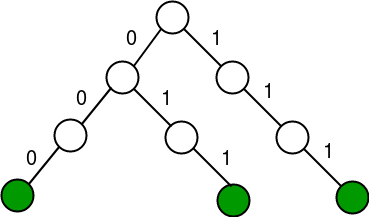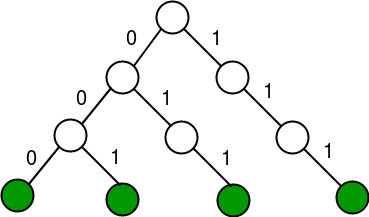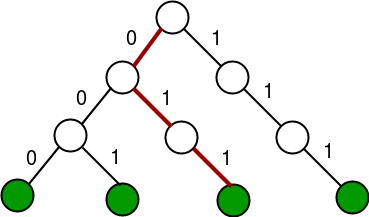Skip to content
Related Articles
Find maximum XOR of given integer in a stream of integers
• Difficulty Level : Medium
• Last Updated : 08 Feb, 2018

You are given a number of queries Q and each query will be of the following types:

1. Query 1 : add(x) This means add x into your data structure.
2. Query 2 : maxXOR(y) This means print the maximum possible XOR of y with all the elements already stored in the data structure.

1 <= x, y <= 10^9
1 <= 10^5 <= Q
The data structure begins with only a 0 in it.

Example:

```Input: (1 10), (1 13), (2 10), (1 9), (1 5), (2 6)
Output: 7 15

Add 10 and 13 to stream.
Find maximum XOR with 10, which is 7
Insert 9 and 5
Find maximum XOR with 6 which is 15.
```

## Recommended: Please try your approach on {IDE} first, before moving on to the solution.

A good way to solve this problem is to use a Trie. A prerequisite for this post is Trie Insert and Search.

Each Trie Node will look following:

```struct TrieNode
{
// We use binary and hence we
// need only 2 children
TrieNode* Children;
bool isLeaf;
};
```

Another thing to handle is that we have to pad the binary equivalent of each input number by a suitable number of zeros to the left before storing them. The maximum possible value of x or y is 10^9 and hence 32 bits will be sufficient.

So how does this work?
Assume we have to insert 3 and 7 into Trie. The Trie starts out with 0 and after these three insertions can be visualized like this:For simplification, the padding has been done to store each number using 3 bits. Note that in binary:
3 is 011
7 is 111
Now if we have to insert 1 into our Trie, we can note that 1 is 001 and we already have path for 00. So we make a new node for the last set bit and after connecting, we get this:Now if we have to take XOR with 5 which is 101, we note that for the leftmost bit (position 2), we can choose a 0 starting at the root and thus we go to the left. This is the position 2 and we add 2^2 to the answer.
For position 1, we have a 0 in 5 and we see that we can choose a 1 from our current node. Thus we go right and add 2^1 to the answer.
For position 0, we have a 1 in 5 and we see that we cannot choose a 0 from our current node, thus we go right.The path taken for 5 is shown above. The answer is thus 2^2 + 2^1 = 6.

 `// C++ program to find maximum XOR in``// a stream of integers``#include``using` `namespace` `std;`` ` `struct` `TrieNode``{``    ``TrieNode* children;``    ``bool` `isLeaf;``};`` ` `// This checks if the ith position in``// binary of N is a 1 or a 0``bool` `check(``int` `N, ``int` `i)``{``    ``return` `(``bool``)(N & (1<isLeaf = ``false``;``    ``temp->children = NULL;``    ``temp->children = NULL;``    ``return` `temp;``}`` ` `// Inserts x into the Trie``void` `insert(TrieNode* root, ``int` `x)``{``    ``TrieNode* Crawler = root;`` ` `    ``// padding upto 32 bits``    ``for` `(``int` `i = 31; i >= 0; i--)``    ``{``        ``int` `f = check(x, i);``        ``if` `(! Crawler->children[f])``            ``Crawler->children[f] = newNode();``        ``Crawler = Crawler->children[f];``    ``}``    ``Crawler->isLeaf = ``true``;``}`` ` `// Finds maximum XOR of x with stream of``// elements so far.``int` `query2(TrieNode *root, ``int` `x)``{``    ``TrieNode* Crawler = root;`` ` `    ``// Do XOR from root to a leaf path``    ``int` `ans = 0;``    ``for` `(``int` `i = 31; i >= 0; i--)``    ``{``        ``// Find i-th bit in x``        ``int` `f = check(x, i);`` ` `        ``// Move to the child whose XOR with f``        ``// is 1.``        ``if` `((Crawler->children[f ^ 1]))``        ``{``            ``ans = ans + (1 << i); ``// update answer``            ``Crawler = Crawler->children[f ^ 1];``        ``}`` ` `        ``// If child with XOR 1 doesn't exist``        ``else``            ``Crawler = Crawler->children[f];``    ``}`` ` `    ``return` `ans;``}`` ` `// Process x (Add x to the stream)``void` `query1(TrieNode *root, ``int` `x)``{``    ``insert(root, x);``}`` ` `// Driver code``int` `main()``{``    ``TrieNode* root = newNode();``    ``query1(root, 10);``    ``query1(root, 13);``    ``cout << query2(root, 10) << endl;``    ``query1(root, 9);``    ``query1(root, 5);``    ``cout << query2(root, 6) << endl;``    ``return` `0;``}`
```7
15
```

The space taken by the Trie is O(n*log(n)). Each query of type 1 takes O(log(n)) time. Each query of type 2 takes O(log(n)) time too. Here n is the largest query number.

Follow up problem: What if we are given three queries instead of two?
1) add(x) This means add x into your data structure (duplicates are allowed).
2) maxXOR(y) This means print the maximum possible XOR of y with all the elements already stored in the data structure.
3) remove(z) This means remove one instance of z from the data structure.
What changes in the Trie solution can achieve this?

This article is contributed by Hemang Sarkar. If you like GeeksforGeeks and would like to contribute, you can also write an article using contribute.geeksforgeeks.org or mail your article to contribute@geeksforgeeks.org. See your article appearing on the GeeksforGeeks main page and help other Geeks.

Please write comments if you find anything incorrect, or you want to share more information about the topic discussed above.

Attention reader! Don’t stop learning now. Get hold of all the important DSA concepts with the DSA Self Paced Course at a student-friendly price and become industry ready.  To complete your preparation from learning a language to DS Algo and many more,  please refer Complete Interview Preparation Course.

In case you wish to attend live classes with industry experts, please refer DSA Live Classes

My Personal Notes arrow_drop_up